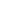#Introduction to Quantitative Social Policy Analysis is a Course

## Introduction to Quantitative Social Policy Analysis

Self-paced

### Full course description

This course introduces you to statistics, with a focus on how they are commonly used for social policy analysis, research, planning, and decision-making. During the first part of this course, you will learn how to use various numerical and graphical tools to describe a single variable, and to summarize the distribution of a variable using measures of central tendency and variability. In addition, you will learn how to use scatterplots and correlation to describe the relationship between a pair of variables. We will also cover introductory concepts of how to sample from a population as well as probability theory.

In the second part of this course, we will cover the basics of “statistical inference” – a set of methods that allows you to draw conclusions about some broader population on the basis of sample data. Most times it is too costly to conduct a pool of the entire population living in the country or even in a city. This is why many institutions, from private firms to the government, commonly question only a sample of the population. But how confident can one be that a group of individuals represents well the entire population of a city, state or country? To address this issue, you will learn how to calculate and interpret confidence intervals (the popular “margin of error”) and how to conduct hypothesis tests based on sample data. You will also learn how to conduct statistical inference on the difference in sample mean(s) and sample proportion(s). Finally, the course will cover how to conduct statistical inference of the relationship between two categorical variables with a two-way table and chi-square statistic as well as the relationship between an explanatory and response variable with simple linear regression. Throughout the course, you will learn how to use the R program to do all of the methods learned.